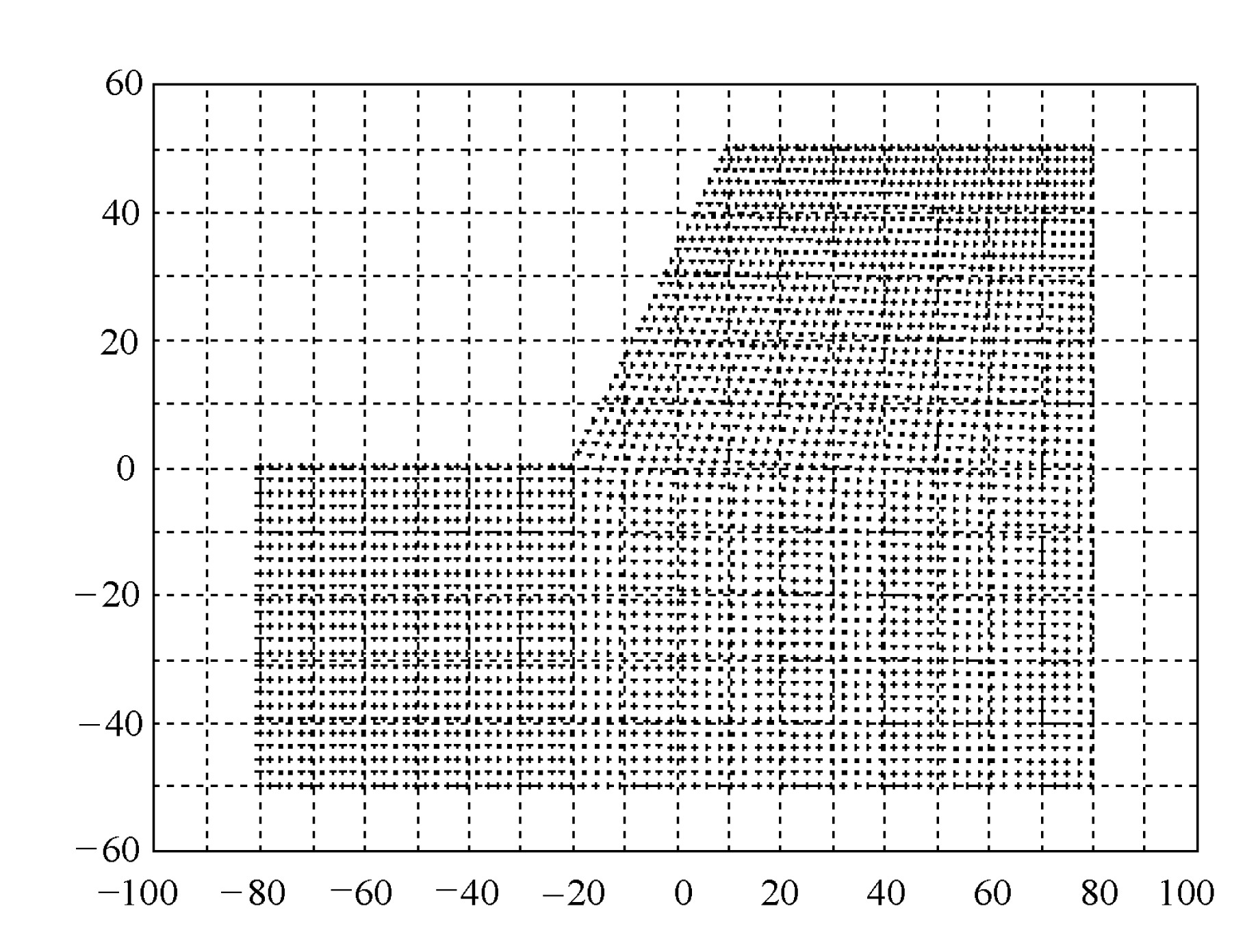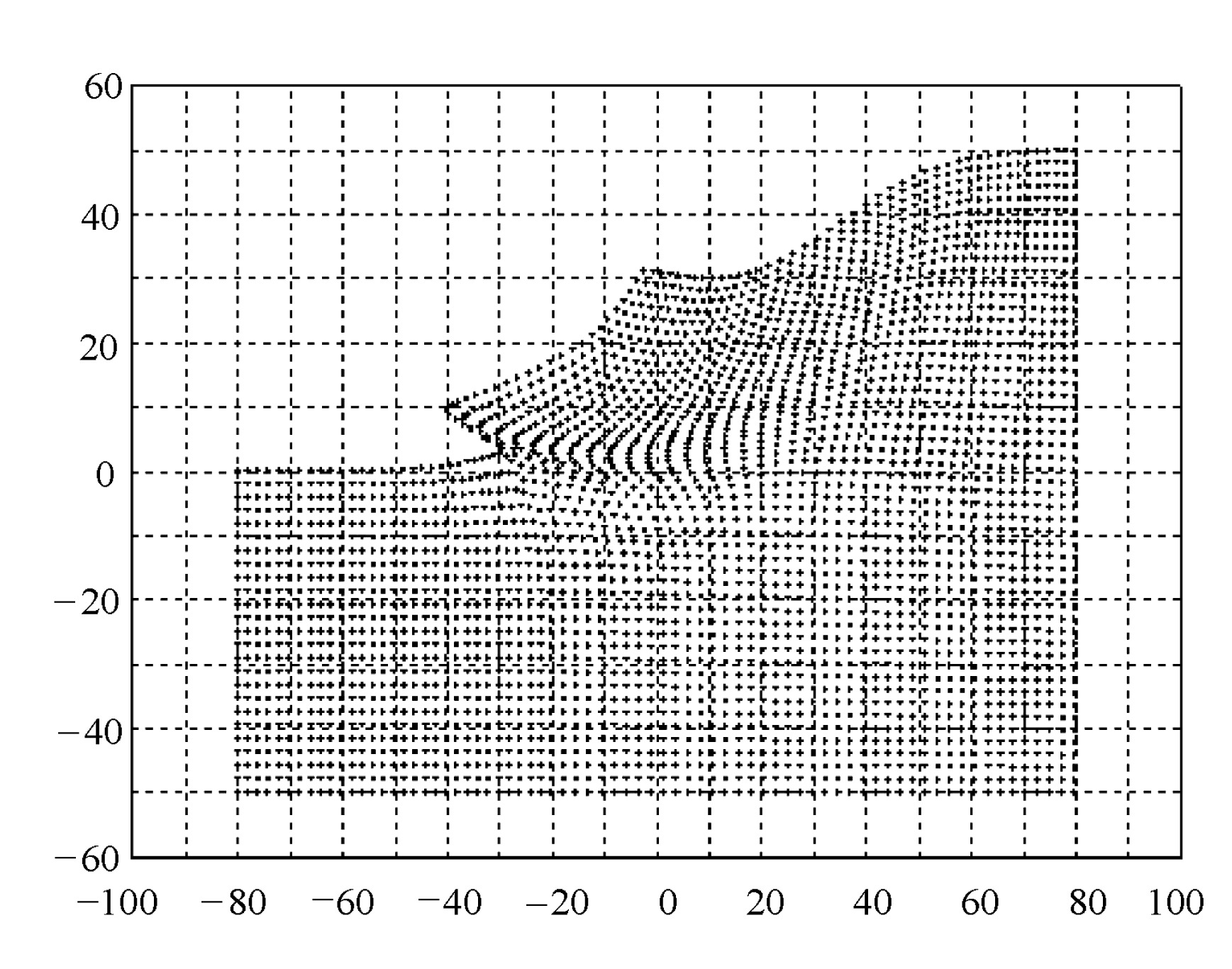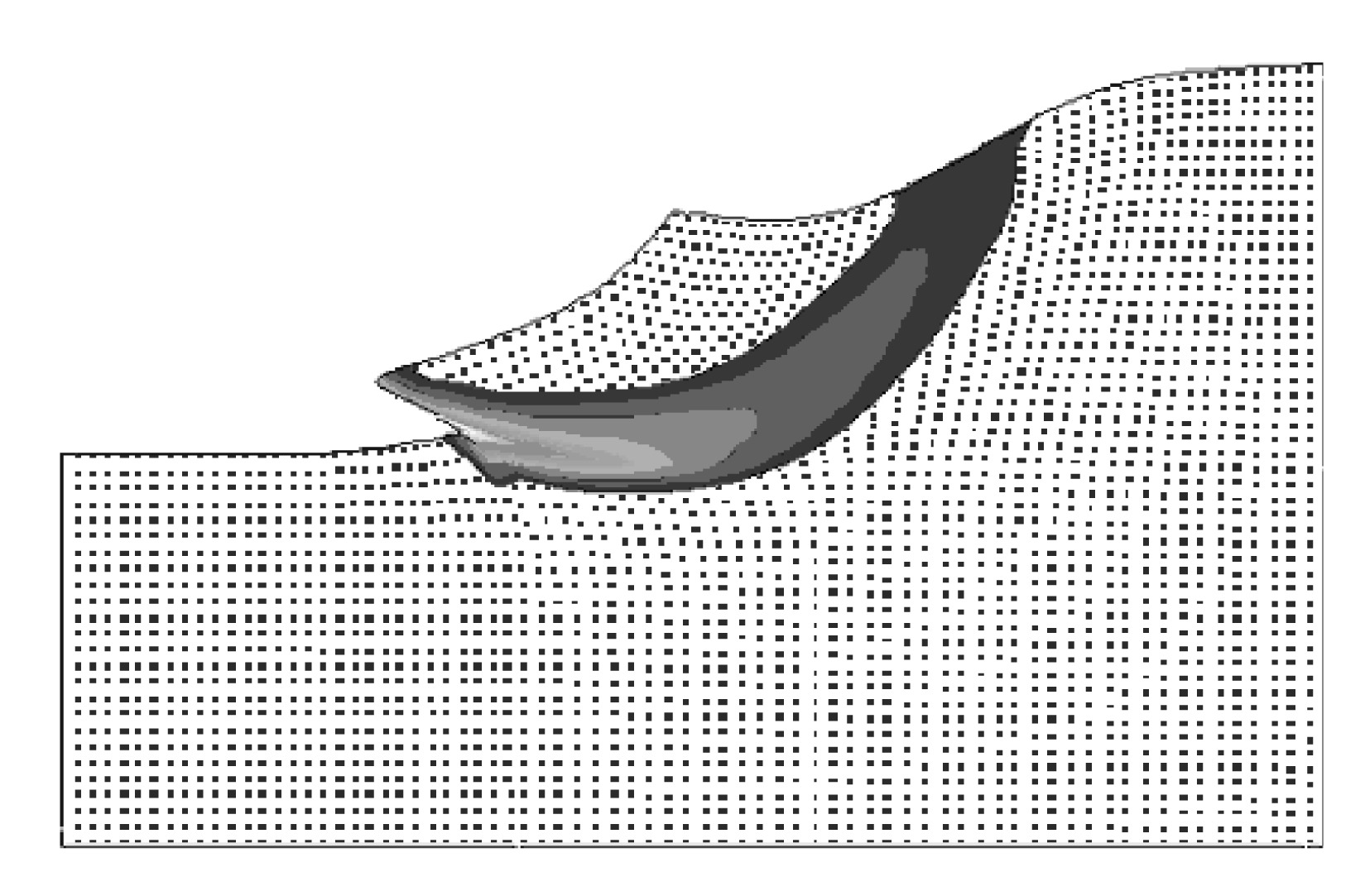Full Text:<3097>

CLC number: O241; TU31

On-line Access:

Revision Accepted: 2004-06-21

Crosschecked: 0000-00-00

Cited: 0

Clicked: 6851

Citations:  Bibtex RefMan EndNote GB/T7714

 Journal of Zhejiang University SCIENCE A 2005 Vol.6 No.2 P.148-154 http://doi.org/10.1631/jzus.2005.A0148

A meshfree method and its applications to elasto-plastic problems

 Author(s):  Ji-fa Zhang1,2, Wen-pu Zhang1,3, Yao Zheng1,2 Affiliation(s):  1. Center for Engineering and Scientific Computation, Zhejiang University, Hangzhou 310027, China; more Corresponding email(s):   jifa_zhang@yahoo.com.cn Key Words:  Meshfree methods, RKPM, Elasto-plasticity, Geotechnical engineering Share this article to： More <<< Previous Article|

ZHANG Ji-fa, ZHANG Wen-pu, ZHENG Yao. A meshfree method and its applications to elasto-plastic problems[J]. Journal of Zhejiang University Science A, 2005, 6(2): 148-154.

@article{title="A meshfree method and its applications to elasto-plastic problems",
author="ZHANG Ji-fa, ZHANG Wen-pu, ZHENG Yao",
journal="Journal of Zhejiang University Science A",
volume="6",
number="2",
pages="148-154",
year="2005",
publisher="Zhejiang University Press & Springer",
doi="10.1631/jzus.2005.A0148"
}

%0 Journal Article
%T A meshfree method and its applications to elasto-plastic problems
%A ZHANG Ji-fa
%A ZHANG Wen-pu
%A ZHENG Yao
%J Journal of Zhejiang University SCIENCE A
%V 6
%N 2
%P 148-154
%@ 1673-565X
%D 2005
%I Zhejiang University Press & Springer
%DOI 10.1631/jzus.2005.A0148

TY - JOUR
T1 - A meshfree method and its applications to elasto-plastic problems
A1 - ZHANG Ji-fa
A1 - ZHANG Wen-pu
A1 - ZHENG Yao
J0 - Journal of Zhejiang University Science A
VL - 6
IS - 2
SP - 148
EP - 154
%@ 1673-565X
Y1 - 2005
PB - Zhejiang University Press & Springer
ER -
DOI - 10.1631/jzus.2005.A0148

Abstract:
Standard finite element approaches are still ineffective in handling extreme material deformation, such as cases of large deformations and moving discontinuities due to severe mesh distortion. Among meshfree methods developed to overcome the ineffectiveness, Reproducing Kernel Particle Method (RKPM) has demonstrated its great suitability for structural analysis. This paper presents applications of RKPM in elasto-plastic problems after a review of meshfree methods and an introduction to RKPM. A slope stability problem in geotechnical engineering is analyzed as an illustrative case. The corresponding numerical simulations are carried out on an SGI Onyx3900 supercomputer. Comparison of the RKPM and the FEM under identical conditions showed that the RKPM is more suitable for problems where there exists extremely large strain such as in the case of slope sliding.

## .  INTRODUCTION

Geotechnical engineering’s well-developed finite element methods (FEMs) for geometrical and material nonlinearities facilitated much of the work accomplished. Nevertheless, standard finite element approaches are still ineffective in handling extreme material deformation, such as cases of large deformations and moving discontinuities due to severe mesh distortion. In order to overcome the ineffectiveness, some FEMs, such as Arbitrary Lagrangian Eulerian (ALE) method, which allow continuous remeshing during computation, were introduced (Hirt, ) and developed (Hughes et al., ; Belytschko et al., ; Donea, ). However, more effort is still required to go around the problem of the oscillation caused by the convective effect (Chen et al., ). To deal with these disadvantages, a new family of numerical methods was developed.

All methods in this family, such as Smooth Particle Hydrodynamics (SPH) (Lucy, ; Monaghan, ), Particle in Cell Methods (PIC) (Harlow, ; Brackbill, ; Sulsky et al., ), Diffuse Element Methods (DEM) (Nayroles et al., ), Element Free Galerkin Methods (EFG) (Belytschko et al., ; Lu et al., ) and Reproducing Kernel Particle Methods (RKPM) (Liu et al., ; ), share a common feature in that no mesh is required and shape functions are formulated based on nodes, which distribute in the domain we want. More detailed classification and overview of meshfree methods, with their advantages and disadvantages, are presented in Fries and Matthies ().

Among these methods, the EFG and the RKPM have been demonstrated as most suitable for structural analysis. Taking into account that the RKPM provides a general formulation for the construction of shape functions for meshfree methods, with specific discretization of the reproduced equation, so that the SPH and the EFG methods can be recovered. We present only the implementation of RKPM in this paper. Chen et al.() extended RKPM to hyper-elasticity with large deformation.

In the present paper, after a brief introduction to the RKPM, we present a meshfree discretization method for elasto-plastic problems with the Drucker-Prager and Mohr-Coulomb models that had been tested and proved suitable for geotechnical materials. A slope stability problem in geotechnical engineering is chosen as a sample case to demonstrate the advantages of the meshfree methods.

## .  REVIEW OF THE RKPM

We begin with the integral transformation of function u(x) by

in which ua(x) is called as the reproduced function of u(x), and the modified kernel function, that is expressed as where a is the dilation parameter of kernel function Φa(xs), and C(x; xs) is called as the correction function, which is constructed to avoid the difficulties resulting from finite domain effects and to minimize the amplitude and phase error. The correction function is expressed by an Nth order polynomial in (xs), that is where

and bi(x) are functions of x, which are determined through satisfying the reproducing conditions. From Taylor series expansion, one can obtain (7) where Finally, the modified kernel function is obtained as where The discretized reproducing equation is obtained by performing numerical integration in Eq.(1). By discretizing the reproducing equation with the trapezoidal rule, we get:

and where n is the total number of particles, and can be interpreted as the shape functions of ua(x). In principle, we take Φa(xxi) to be positive and set its maximum value at x=xi. The function should rapidly approach zero after |x=xi| exceeds a small value so that the shape function associated with node i has an influence on only a small group of surrounding nodes to provide computational efficiency.

The extension of Φa to a multi-dimensional case can be achieved by using tensor products of the one-dimensional kernel functions

In this construction, the supports of kernel functions are rectangular and hexahedral in geometries, each with a center xi and dimensions 2a1×2a2 and 2a1×2a2×2a3, in two- and three-dimensional problems, respectively.

The correction function for the multi-dimensional case is expressed as where The coefficients are determined from the reproducing conditions. Finally, the multi-dimensional RKPM interpolation is obtained as where

and ΔVi is the volume associated with particle i.

## .  RKPM FOR ELASTO-PLASTIC PROBLEMS

Different from those in the FEM, the shape functions in the RKPM do not possess the Kronecker delta properties, i.e., . This will cause difficulties in numerical implementation while dealing with kinetic constraints on the geometrical boundaries of a model. A modified RKPM shape function that possesses Kronecker delta properties is required for numerical implementation.

Recall that where By substituting Eq.(16) into Eq.(15), one can obtain where Note that

and

are nodal values. ua and δua satisfy the following boundary conditions: where denotes a particle numbers set in which the associated particles are located on From Eq.(18), we directly obtain:

By using the expressions obtained above, we can discretize the weak forms of the elasto-plastic problems. As we known well, the equilibrium equation of the small strain elasto-plastic problem can be written as

Its weak form based on virtual displacement principle can be written as

with the constitutive relations or

Similar to those in finite element methods, the following expressions must hold theoretically

in which BT and are defined as u= δu= ε= δε= respectively. B has a well known relation with as

in which L is a differential operator between strains and displacements. However, different from that in finite element methods, volume integration of Eq.(25) over the domain Ω is not the sum of the individual elements, but the points.

In addition, though Eq.(25) hold theoretically, it will not generally be satisfied at any stage of numerical computation, owing to the nonlinearities of elasto-plastic problems. Namely, the residual force vector is

for evaluating the material tangent stiffness matrix.

At any stage, the incremental form of Eq.(25) has to be employed. This implies that, for an increment of load, we have

Based on this equation, the numerical procedure, such as Newton-Raphson Method and Tangential Stiffness Method (sometimes named as Generalized Newton-Raphson Method), can be directly applied.

## .  CASE STUDY

For the frictional materials such as soil and rock in geotechnical engineering, a non-associated or associated Drucker-Prager (and Mohr-Coulomb) plasticity model is appropriate for modeling its constitutive behavior. This can be described generally as follows (Drucker and Prager, ).

A quadratic stored energy function Ψ(εe, ξ), which results in linear elasticity and linear hardening, is defined as where ce and H are constant modulus tensors. Note that Ψ(εe, ξ) could be defined such that nonlinear elasticity and/or hardening would have resulted. The fourth-order tensor ce is a tensor of isotropic elastic tangent modulus defined as where is the elastic bulk modulus, and are the Lame parameters, (1)ij=δij is the Kroneker delta, and (I)ijkl=(δikδji+δilδjk)/2 is the fourth-order identity tensor.

Let the strain-like vector of plastic internal variables ξ : Ω×[0,T]→(2 have a volumetric component and a deviatoric component where and The hardening/softening modulus matrix H is defined as where and are the bulk and shear hardening/softening modulo, respectively. The stress-like vector of plastic internal variables α is defined as

A Drucker-Prager yield function takes the form

with derivatives where and , in which Note that for the continuum mechanics convention used throughout this paper, compression implies p<0. The above material constants and β may be defined in terms of the cohesion and friction angle used to describe a Mohr-Coulomb material (Owen and Hinton, )

The value A=−1 coincides with a cone that circumscribes the Mohr-Coulomb envelope−passing through its outer apexes−in three-dimensional stress space, and A=1 coincides with a cone that passes through the inner apexes of the Mohr-Coulomb envelope. Because of the form of in Eq.(34), standard bulk and shear hardening/softening through α1 and α2 cause the size of the yield cone to change, and not its shape. Incorporating additional hardening/softening through β would allow frictional hardening/softening that is appropriate for modeling a cohesionless granular material, such as sand, thus the change of slope of the yield cone would be possible.

A plastic potential function φ(σ, α) is defined similar to the yield function ϕ(σ, α) as

with derivatives where b is the material dilation constant. Associated plasticity results if β=b, but typically for soil and rock, this is not the case. Usually there exists β>b with b>0 for a dilatant material, and b<0 for a contractant material. By setting β=b=0, the J2 flow (von Mises) plasticity model is recovered, which is useful for modeling the undrained conditions in a cohesive soil.

With the plastic potential function φ(σ, α) as defined in Eq.(36), the evolution of ξ then becomes

Note that b takes the form

which is analogous to the dilatancy factor used by Rudnicki () and Rudnicki and Rice ().

There are many kernel functions proposed. Here we employ a cubic spline function as the kernel function of Eq.(11) where

As an illustrative case, we study a plane strain problem of slope stability in geotechnical engineering. The geometrical model of the slope is shown as Fig.1 with a slope of 5/3 and is discretized with 3029 points (Fig.3). The constitutive relation is Mohr-Coulomb model. Under self-gravity, the slide will happen while the friction and cohesive strength of soil decreases.Fig.1
Geometrical model with a grid showing coordinates

During the numerical computation, the cubic spline function is chosen as kernel function Eq.(40), with the cohesive-like strength parameter of 0.015, friction-like parameter of 0.04, dilation and hardening parameter of zero. As a result, the maximum relative and maximum absolute errors are 10−10 and 10−12, respectively. The deformed shape of the slope is depicted in Figs.4 and 6. In order to compare with mesh-based methods, such as the finite element method, the computed results by the meshfree method is shown with and without a mesh as Figs.5 and 2, respectively. The extremely deformed part near the slope-toe is outlined with a mesh in Figs.7 and 8.Fig.2
Deformed shape with a grid showing coordinatesFig.6
Deformed shape showing strain energy density

## .  CONCLUSION

The numerical computation shows that, while the deformation is not sufficiently large, such as in an elastic stage, the FEM and the RKPM will produce almost the same results, but the meshfree method costs more computation time. However, while the deformation is large enough, even with the same discretization and allowable errors, the FEM would result in divergence with RKPM resulting in convergence. As to the situation of extreme deformation, for example the case of slope sliding we are discussing here, the FEM cannot deal with it due to the non-positiveness of the Jacobian, which is caused by the severe mesh distortion. The conclusion can be drawn that for extreme deformation, the meshfree method RKPM is more appropriate.

In addition, it should be mentioned that parallel computation was used for complete comparison between FEM and RKPM on an SGI Onyx3900 supercomputer with 1, 2, 4, 8 and 16 processors utilized.

## ReferencesBelytschko, T., Flanagan, D.P., Kennedy, J.M., 1982. Finite element methods with user-controlled meshes for fluid-structure interaction. Comput Methods Appl Mech Engrg, 33:669-688.Belytschko, T., Lu, Y.Y., Gu, L., 1994. Element-free galerkin methods. Int J Numer Methods Eng, 37:229-256.Brackbill, J.U., 1986. A method for adaptive zoned, particle-in-cell calculation of fluid flows in two dimensions. J Comput Phys, 65:314-343.Chen, J.S., Pan, C., Wu, C.T., Liu, W.K., 1996. Reproducing kernel particle methods for large deformation analysis of non-linear structures. Comput Methods Appl Mech Engrg, 139:195-227.Chen, J.S., Pan, C., Wu, C.T., 1997. Large deformation analysis of rubber based on a reproducing kernel particle method. Comp Mech, 19:211-227.Donea, J., 1983. Arbitrary Lagrangian-Eulerian Finite Element Methods. Computational Methods for Transient Analysis, Elsevier, Amsterdam,:474-515.Drucker, D.C., Prager, W., 1952. Soil mechanics and plastic analysis or limit design. Q Appl Math, 10:157-165.Fries, T., Matthies, H.G., 2003. Classification and Overview of Meshfree Methods. , Report of Technical University Braunschweig, :Harlow, F.H., 1964. Particle-in-cell computing method for fluid dynamics. Methods for Comput Phys, 3:319-343.Hirt, C.W., Amsden, A.A., Cook, J.L., 1974. An arbitrary Lagrangian-Eulerian computing method for all flow speeds. J Comput Phys, 14:227-253.Hughes, T.J.R., Liu, W.K., Zimmermann, T.K., 1981. Lagrangian-Eulerian finite element formulation for incompressible viscous flows. Comput Methods Appl Mech Engrg, 29:329-349.Liu, W.K., Jun, S., Li, S., Adee, J., Belytschko, T., 1995. Reproducing kernel particle methods for structural dynamics. Int J Numer Methods Eng, 38:1655-1679.Liu, W.K., Li, S., Belytschko, T., 1997. Moving least square reproducing kernel methods: (I) methodology and convergence. Comp Methods Appl Mech Eng, 143:113-154.Lu, Y.Y., Belytschko, T., Gu, L., 1994. A new implementation of the element free Galerkin method. Comp Methods Appl Mech Eng, 113:397-414.Lucy, L.B., 1977. A numerical approach to the testing of the fission hypothesis. The Astronomical Journal, 82(12):1013-1024.Monaghan, J.J., 1982. Why particle methods work. SIAM J. Sci.−. Stat Compt, 3(4):422-433.Nayroles, B., Touzot, G., Villon, P., 1992. Generalizing the finite element method: diffuse approximation and diffuse elements. Comp Mech, 10:307-318.Owen, D.R.J., Hinton, E., 1980. Finite Elements in Plasticity: Theory and Practice. , Pineridge, Swansea, 215-237. :215-237.Rudnicki, J.W., 1977. The inception of faulting in a rock mass with a weakened zone. J Geophys Res, 82:844-854.Rudnicki, J.W., Rice, J.R., 1975. Conditions for the localization of deformation in pressure-sensitive dilatant materials. J Mech Phys Solids, 23:371-394.Sulsky, D., Chen, Z., Schreyer, H.L., 1994. A particle method for history-dependent materials. Comput Methods Appl Mech Engrg, 118:179-196.

<1>

Daniel@University of Florida<kjha001@fiu.edu>

2013-07-21 13:49:32

Very good